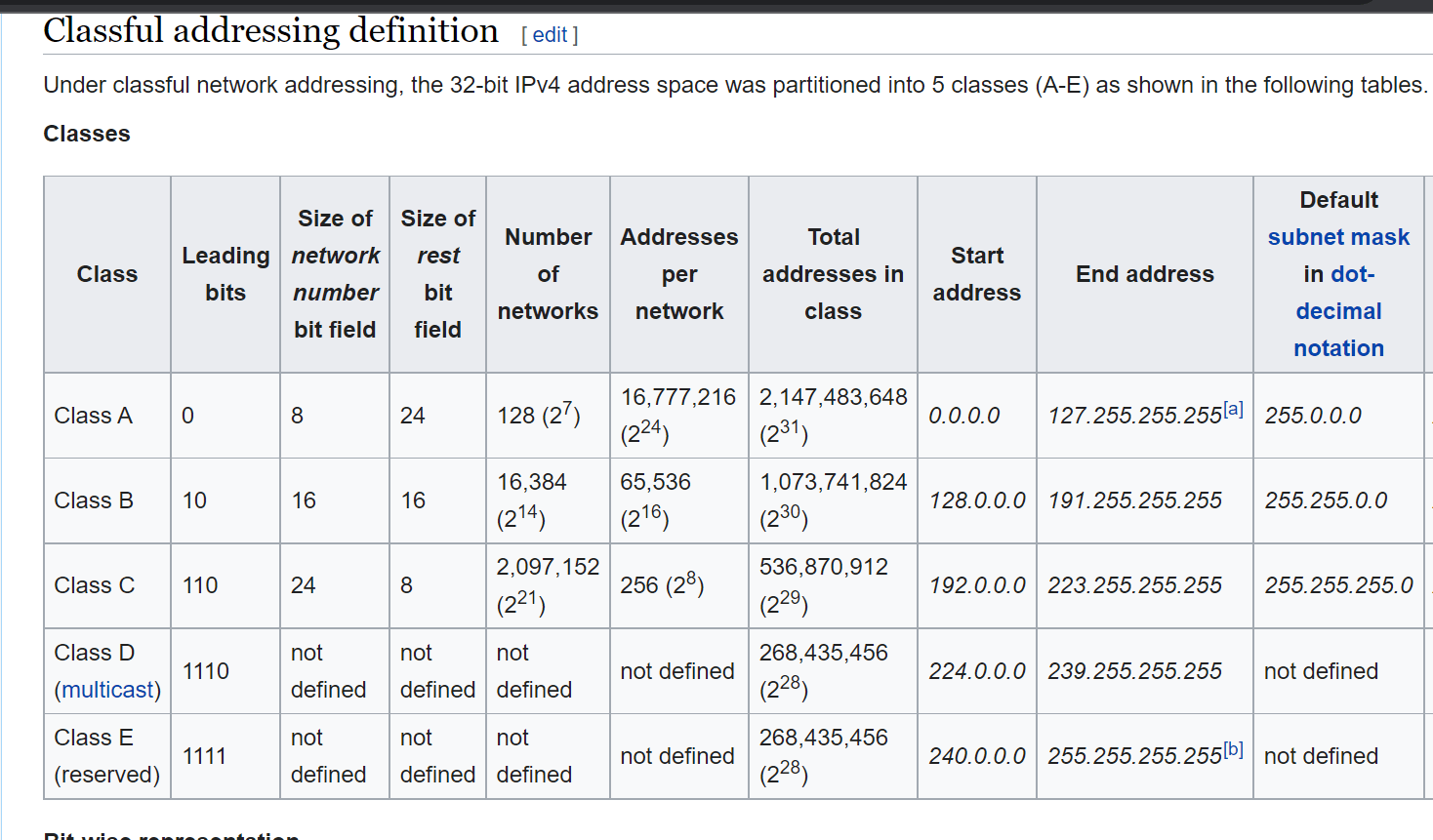## Networking Contd….

• If we consider only octets for network creations we have only few possibilities
• 255.255.255.0 => 2^8-2 ~= 250
• 255.255.0.0 => 2^16-2 ~= 65000
• 255.0.0.0 => 2^24-2
• To Create networks, the older approach was to use Classful Networking.
• Class A
• Class B
• Class C
• Class D
• Class E• Lets consider subnet mask as a binary number
``````ip:   192.168.0.4
sm:   255.255.255.0
11111111.11111111.11111111.00000000
``````
• Now lets assume i want to create a network of size 500
``````2^n ~= 500
n (number of zeros) = 9
N (number of ones) = 32-9 = 23
sm: 11111111.11111111.11111110.00000000
``````
• Lets find a subnet mask for a network of size 22
``````2^n ~= 22
n (number of zeros) = 5
N (number of ones) = 32-5 => 27
sm: 11111111.11111111.11111111.11100000
255.255.255.224

x.x.x.x/27

``````
• Lets find a subnet mask for
• 1,20,000 devices
``````2^n ~= 120000
n = 17
N = 32-17 = 15
SM: 11111111.11111110.00000000.00000000
255.254.0.0
x.x.x.x/15
``````
• 10 devices
``````2^n ~= 10
n = 4
N = 32-4 = 28
SM: 11111111.11111111.11111111.11110000
255.255.255.240
x.x.x.x/28
``````
• 5000 devices
``````2^n ~= 5000
n = 13
N = 32 -13 = 19
SM: 11111111.11111111.11100000.00000000
255.255.224.0
x.x.x.x/19

``````
• CIDR Notation:
``````x.x.x.x/N
N = number of ones in subnet mask
``````
• Lets expand a cidr notation
``````192.168.0.0/24
SM: 11111111.11111111.11111111.00000000

range => 192.168.0.0 - 192.168.0.255

192.168.0.0/22
SM: 11111111.11111111.11111100.00000000
192.168.0.0 to 192.168.3.255

10.0.0.0/12
SM: 11111111.11110000.00000000.00000000
10.0.0.0 to 10.15.255.255

``````

This site uses Akismet to reduce spam. Learn how your comment data is processed.## About continuous learner

devops & cloud enthusiastic learner